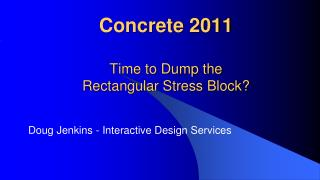DownloadDownload PresentationConcrete 2011 Time to Dump the Rectangular Stress Block?

# Concrete 2011 Time to Dump the Rectangular Stress Block?

Télécharger la présentation## Concrete 2011 Time to Dump the Rectangular Stress Block?

- - - - - - - - - - - - - - - - - - - - - - - - - - - E N D - - - - - - - - - - - - - - - - - - - - - - - - - - -
##### Presentation Transcript

1. Concrete 2011Time to Dump the Rectangular Stress Block? Doug Jenkins - Interactive Design Services

2. Introduction Everything should be made as simple as possible, ... but not simpler.Albert Einstein

3. Introduction • Are the rectangular stress block provisions for the calculation of ultimate bending capacity “too simple”? • It depends

4. Background Eurocode 2 Rectangular Stress Block

5. Background • All the major international concrete codes have provisions for a rectangular stress block. • For concrete strengths up to 50 MPa the AS 3600 provisions are almost identical to those in ACI 318. • The current AS 3600 has provisions for a reduced stress factor for concrete strengths above 50 Mpa, whereas ACI 318 uses a constant stress factor, and has no specified upper limit on concrete strength. • Eurocode 2 has provisions for rectangular, parabolic-linear, and non-linear stress blocks

6. Rectangular Block Advantages • Simplicity • Well-validated, experimentally and in practice • Good agreement with more complex stress blocks for rectangular cross sections.

7. Rectangular Block Disadvantages • Significant error in calculated moment capacity for non-rectangular sections • Adjustment of stress factor required for concrete strengths greater than 50 MPa • Alternative stress blocks allow the same provisions to be applied for all concrete strengths and cross sections without an associated loss of accuracy

8. Alternative Stress Blocks

9. Equivalent Stress Block Calculation • Find factors α (stress factor) and γ (depth factor) such that the area under the rectangular stress block and the lever arm about the neutral axis are equal to the equivalent values for a curvilinear stress block: • Find the lever arm of the curvilinear stress block for unit stress block depth, LAc • Find γsuch that the rectangular stress block lever arm, Lac, is equal to LAc: • (1 – γ/2) = Lac • γ = 2(1 – Lac)

10. Equivalent Stress Block Calculation • Find α such that the area under rectangular stress block is equal to the area under the curvilinear stress block:

11. Equivalent Stress Block Parameters

12. Equivalent Stress Block Parameters

13. Alternative Stress Block Comparison • Calculations in accordance with AS 3600 Cl. 8.1.2 • No capacity reduction or partial safety factors applied • Cylinder strength factored by 0.9, in accordance with AS 3600 • Reinforcement : elastic-plastic stress-strain curve, yield stress =500 MPa, elastic modulus =200 GPa. • Section ultimate bending capacity calculated about the centroid of the gross concrete section • Ultimate strain adjusted to maximise capacity • Three sections compared at 32 MPa, 65 MPa and 90 MPa

14. Alternative Stress Block Comparison

15. Rectangular Section; 32 MPa

16. Rectangular Section; 90 MPa

17. Circular Section; 90 MPa

18. I Section; 90 MPa

19. General Conclusions • Eurocode 2 parabolic/rectangular block (EC2-P) consistent with non-linear curves, and more conservative for high strength grades, used as benchmark. • “Equivalent rectangular stress block” (Equ-R) results were almost identical to the EC2-P block for rectangular cross sections. • Equ-R results up to about 10% unconservative with a circular section, or 10% conservative with an I section. • ACI 318 results very unconservative with 90 MPa concrete.

20. General Conclusions • Eurocode 2 rectangular stress block (EC2-R) did not correlate well with EC2-P results: least conservative at 32 MPa and most conservative at 90 MPa. • AS 3600 rectangular stress block results similar to EC 2 equivalent stress block. • Eurocode 2 recommends reduction of concrete stress by 10% when width of compression zone reduces towards the compression face.

21. Recommendations • Retain rectangular stress block for compatibility with existing software and design charts, but: • Introduce a parabolic-rectangular stress block with similar form to Eurocode 2. • Provide simple procedure for deriving parameters for an equivalent rectangular stress block for use with rectangular compression zones. • Recommend parabolic-rectangular block for non-rectangular sections with significant axial load. • Review Eurocode parameters for concrete grades 80 MPa and higher.

22. Further Information and Software • http://newtonexcelbach.wordpress.com/